# Bottle Rocket Model

• I
Gold Member
Edit: I've been catching errors I've made in the transcribing the formulas into latex ( not errors I made in the actual model..yet ). There may be more. I did this in one sitting.

I've been trying to model a bottle rocket (water propellant, compressed air fuel) and my model seems ok for a typical 2L soda bottle type dimension, but when I decrease the radius of the nozzle, I expect there to be a "maximum altitude" and then a decrease to "no altitude" for some small radius, but the model is showing otherwise. I can see that the velocity of the propellant goes right through the speed of sound as I decrease the nozzle radius, and I think this is the problem. I was hoping the shear force in the nozzle would prevent this issue, but it isn't doing the job. Below is the model (its a bit involved). I've tried to make sure I've explained it, but If I miss things, please let me know (that's why I'm posting it).

Figure 1 is the initial setup: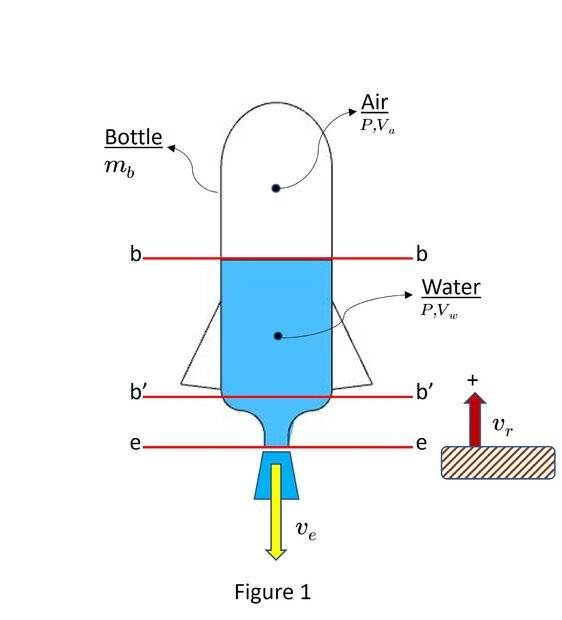Initially there is some volume of air ## {V\llap{-}}_a## at a pressure ## P_o ## (gauge pressure). The volume of propellant ( water ) is ## { V\llap{-} }_w ## . Then at ## t = 0 ## the rocket is released. The velocity of the rocket ## v_r ## is w.r.t. a stationary frame fixed to the ground, and ## v_e ## is the velocity of the jet w.r.t. the rocket.

Figure 2 is the Free Body Diagram of the external forces acting on the rocket.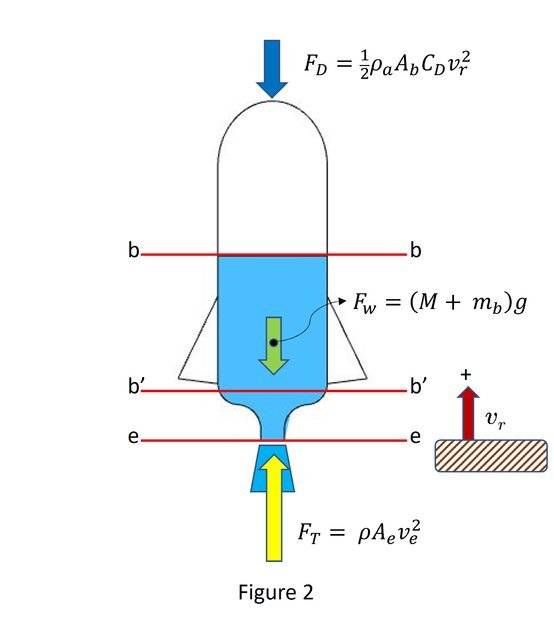Section 1 - Applying Newton 2nd Law:

Assuming:

the weight of the air compressed air is negligible.
The fluid jet exits at ## P_{atm} ## (valid for fluid jet velocity that is less than the local speed of sound in the fluid)

$$M_r \frac{d v_r}{dt} = -\dot{M} v_e - M_r g - \frac{1}{2} \rho_a A_b C_D v_r^2$$

From here change independent variable from time ## t ## to mass of the propellant ##M## by noticing that:

$$M_r = M + m_b \implies \dot{M_r} = \dot{M} = - \rho A_e v_e$$

Applying the Chain Rule:

$$- M_r \rho A_e v_e \frac{d v_r}{d M_r} = \rho A_e v_e^2 - M_r g - \frac{1}{2} \rho_a A_b C_D v_r^2 \tag{1}$$

Section 2- ## v_e ## of Propellant:

Figure 3 shows a Force and Momentum diagram applied to the control volume shown by the dashed boundary.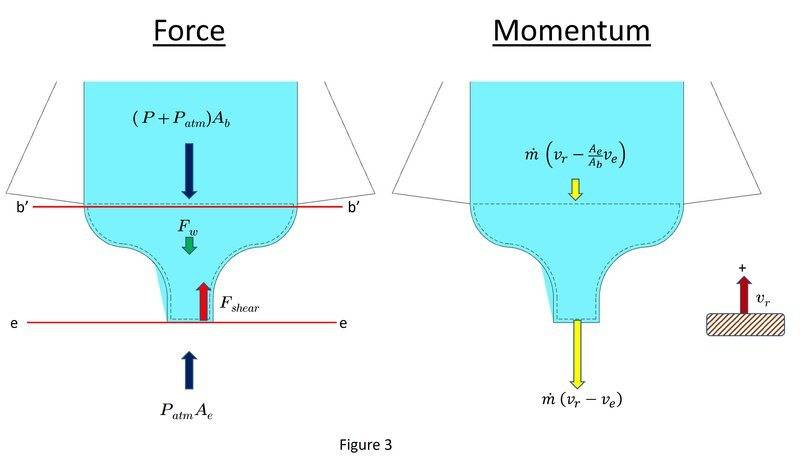From the Reynolds Transport Theorem

$$\sum F = \frac{d}{dt} \int_{cv} \rho \mathbf{v} dV\llap{-} + \int_{cs} \mathbf{v} \rho \mathbf{V} \cdot d \mathbf{A}$$

Assuming:
Uniform velocity across sections b'-b' and e-e
Continuity
The fluid in the control volume is incompressible

$$\sum F = m_{cv} \frac{d v_r}{dt} + \dot{m} v_{ee} - \dot{m} v_{b'b'}$$

Furthermore the mass in the control volume can basically be taken to be very small and I neglected the accumulation term.

$$\sum F = \dot{m} \left( v_r - v_e \right) - \dot{m} \left( v_r - \frac{A_e}{A_b} v_e \right)$$

or substituting for ## \dot{m} ##

$$\sum F = \rho A_e \left( \frac{A_e}{A_b} - 1 \right) v_e^2$$

Force Balance:

Assuming:

Weight of mass in the control volume is negligible
Pressure in section b-b is equal to b'-b'
1. No pressure loss from viscous effects in this section
2. No pressure increase from hydrostatic pressure in the column
3. No pressure increases from inertial forces associated with ## \frac{dv_r}{dt} ##

$$\sum F = P_{atm} A_e - \left( P + P_{atm} \right) A_b - \pi D l \mu \left. \frac{dv}{dy} \right|_{r_o}$$

The last term on the right is the shear force, and the minus sign comes from the sign convention ( I think it correct ).

To get a functional slope ## \frac{dV}{dy}## at the boundary I had to assume a parabolic velocity distribution. *I'm aware that this somewhat contradicts an earlier assumption in applying the Reynolds Transport Theorem where I assumed uniform velocity distribution across the nozzle ( section e-e ).

Figure 4 shows this distribution.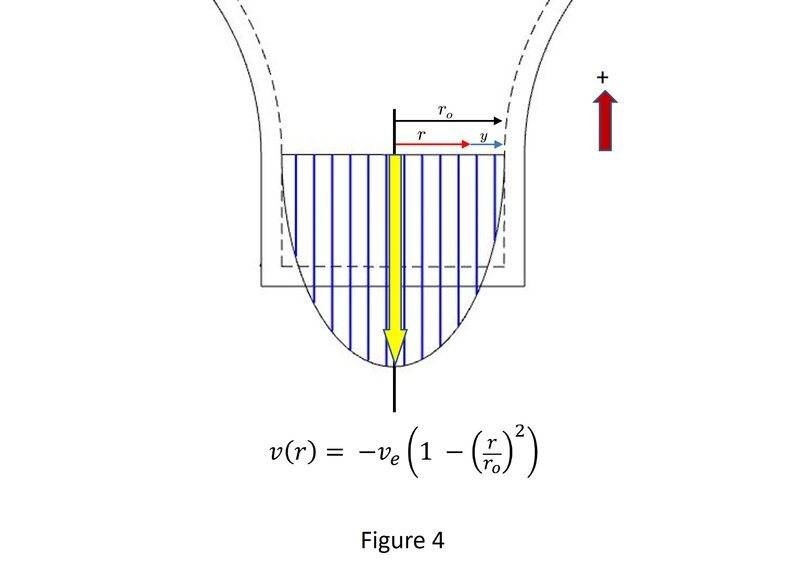from this we have that:

$$\left. \frac{dv}{dr} \right|_{r_o} = 2 v_e \implies \left. \frac{dv}{dy} \right|_{r_o} = -2 v_e$$

Substiting that result back in:

$$\sum F = P_{atm} A_e - \left( P + P_{atm} \right) A_b + 4 \pi l \mu r_e v_e$$

Where:
## P ## is the gauge pressure of the compressed air
## \mu ## is the viscosity
## r_e ## is the exhaust radius

putting Section 2 all together:

$$\rho A_e \left( \frac{A_e}{A_b} - 1 \right) v_e^2 = P_{atm} A_e - \left( P + P_{atm} \right) A_b + 4 \pi l \mu r_e v_e \tag{2}$$

Then solve the quadratic for ## v_e ##

$$v_e = \frac{-b - \sqrt{ b^2 - 4 a c } }{ 2 a}$$

Where:

## a = \rho A_e \left( \frac{A_e}{A_b} - 1 \right) ##

## b = - 4 \pi l \mu r_e ##

## c = \left( P + P_{atm} \right) A_b - P_{atm} A_e ##

Section 3 - Isothermal Expansion of the Air

The last step before (1) is able to be numerically solved is to determine the pressure of the gas as a function of the mass of water in the bottle.

$$\left( P_o + P_{atm} \right) {V\llap{-}_a}_o = \left( P + P_{atm} \right) { V\llap{-} }_a$$

Solving for ## P ##

$$P = \left( P_o + P_{atm} \right) \frac{ {V\llap{-}_a}_o }{ V\llap{-}_a } - P_{atm} \tag{3}$$

Furthermore, we have that the total volume of the bottle ## V\llap{-} ## is fixed such that:

$$\rho V\llap{-}_a = \rho V\llap{-} - M$$

Multiply the term on the left in (3) by ## \frac{\rho}{\rho} ##

$$P = \left( P_o + P_{atm} \right) \frac{ \rho {V\llap{-}_a}_o }{ \rho V\llap{-} - M } - P_{atm} \tag{4}$$

So, you put that all together and solve (1) numerically for the EOM during powered flight ## v_r (M) ##.

To calculate the peak altitude:

its the powered flight altitude + the altitude from flight at burnout.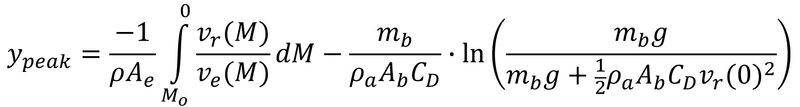Getting this all down might have been more work than its worth... If I need to elaborate on my variables, derivations more let me know. It might take me a while to get the latex to parse so its readable

Thanks for any help, comments ect.. on what might be incorrect.

Last edited:
•Lnewqban

Homework Helper
Gold Member
•berkeman
Gold Member
http://cjh.polyplex.org/rockets/simulation/
Thanks for the link. My latest model is over-shooting it pretty significantly, and the simulation includes the second stage (compressed gas exiting the nozzle). So, I'm a bit worried. However, I can't determine the model behind the simulation, so I'm not sure how it was derived. There are several ways to approach this ( I have many different models now ). I just though this one should be used. I might post some other ways with less "true" assumptions, but are easier to work with.

Last edited:
•Lnewqban
So unfortunately I don't have a lot of time right now so I don't have time to go through your full derivation. However, I did write almost exactly that code for a college class back in 2009 (damn, has it really been that long?), so I have a pretty good idea how it should work and where you might be going wrong (and my code matched measurements of both the flight tests and static tests we performed quite well). Purely based on knowing what works well for this kind of simulation, here are my suggestions.

First off, I'd skip the parabolic distribution for now. That's adding unnecessary complexity, and likely isn't even that accurate since you don't have a long pipe section at the nozzle to allow the flow profile to fully develop. Honestly, I'd just ignore wall friction entirely until you have a simpler code working.

Second, since water is incompressible (at any reasonable pressure and velocity you'll see here), you don't need to go through all the calculus above. You can simply use the bernoulli relation to find exit velocity, since you know the pressure and velocity inside the bottle and you know the pressure at exit (ambient, as you said). You can then use the exhaust velocity to find mass flow rate (based on exit diameter) and then the thrust.

Third, the expansion in the bottle as the water leaves is much better modeled as adiabatic rather than isothermal. Isothermal is a good model for slow processes where it has time to equilibriate with the surroundings, but this is a pretty fast process and there isn't much heat transfer.

I'd start with a model based on the above and see if that gets you closer to something more reasonable, and after that you can start to add in extra complexities. One interesting extra complexity for example is that depending on the ratio of water to air volume at the beginning and the pressure, there can be substantial residual pressure left when the water runs out. If this is the case, there's a not-insignificant very short duration "pop" of additional impulse that happens as this air accelerates out the bottle, and you can even end up with a briefly supersonic flow if the residual pressure is high enough. This isn't a lot of impulse, but it occurs when the rocket is lightest, so it's actually a pretty large source of error in some cases if ignored. Ultimately you probably also want to include aerodynamic drag if you want to accurately model a flight, but that's entirely separate from modeling the propulsion, so I would wait to do a full flight simulation model until you can get the propulsion model reasonably close.

•erobz and Ibix
Gold Member
So unfortunately I don't have a lot of time right now so I don't have time to go through your full derivation. However, I did write almost exactly that code for a college class back in 2009 (damn, has it really been that long?), so I have a pretty good idea how it should work and where you might be going wrong (and my code matched measurements of both the flight tests and static tests we performed quite well). Purely based on knowing what works well for this kind of simulation, here are my suggestions.

First off, I'd skip the parabolic distribution for now. That's adding unnecessary complexity, and likely isn't even that accurate since you don't have a long pipe section at the nozzle to allow the flow profile to fully develop. Honestly, I'd just ignore wall friction entirely until you have a simpler code working.

Second, since water is incompressible (at any reasonable pressure and velocity you'll see here), you don't need to go through all the calculus above. You can simply use the bernoulli relation to find exit velocity, since you know the pressure and velocity inside the bottle and you know the pressure at exit (ambient, as you said). You can then use the exhaust velocity to find mass flow rate (based on exit diameter) and then the thrust.

Third, the expansion in the bottle as the water leaves is much better modeled as adiabatic rather than isothermal. Isothermal is a good model for slow processes where it has time to equilibriate with the surroundings, but this is a pretty fast process and there isn't much heat transfer.

I'd start with a model based on the above and see if that gets you closer to something more reasonable, and after that you can start to add in extra complexities. One interesting extra complexity for example is that depending on the ratio of water to air volume at the beginning and the pressure, there can be substantial residual pressure left when the water runs out. If this is the case, there's a not-insignificant very short duration "pop" of additional impulse that happens as this air accelerates out the bottle, and you can even end up with a briefly supersonic flow if the residual pressure is high enough. This isn't a lot of impulse, but it occurs when the rocket is lightest, so it's actually a pretty large source of error in some cases if ignored. Ultimately you probably also want to include aerodynamic drag if you want to accurately model a flight, but that's entirely separate from modeling the propulsion, so I would wait to do a full flight simulation model until you can get the propulsion model reasonably close.
Yeah, My first round draft was using "The Energy Equation" , its basically Bernoulli's Equation repackaged to include viscous effects:

Using the diagrams of the latest model it is as follows:

$$\frac{P_{b}}{ \gamma} + \frac{v_{b}^2}{2g} = \frac{v_e^2}{2g} + k\frac{v_e^2}{2g} + \frac{2 f l}{r_e} \frac{v_e^2}{2g}$$

Where:
## k ## is a contraction loss coefficient
## \frac{2 f l}{r_e}## characterizes the loss through the "pipe" of radius ## r_e##

This leads to a more simple:

$$v_e^2 = \frac{2}{\rho} \left( \frac{1}{ 1 + k + \frac{2 f l}{r_e} - \left( \frac{r_e}{r_b} \right)^4 } \right) P$$

However, I abandoned it because it is derived in an inertial frame (like Bernoulli's Equation), and the process is happening in a non-inertial frame. So I decided to take a step backward ( really 2) to the "next closest " first principle in which a "non-inertial frame" can be accounted for without too much difficulty ( i.e. The Momentum Equation ), thus far seemingly without success...

As for the adiabatic expansion, good point! Thank You. I'll re formulate that part, but it shouldn't correct the issue of the jet velocity passing through the sonic barrier ( and perhaps blow right by ) as the nozzle is decreased. I was counting on friction to stop that from happening. Maybe it does though, I'll work on it.

As for the "Pop" as the second stage initiates . I looked at that, but that becomes massively complex very quickly with compressed gas near sonic regime.

It could be that I've just bungled the application of the equation because of the reference frame issue. Thats why I was hoping some physicist could set me on the right track!

I did write almost exactly that code for a college class back in 2009 (damn, has it really been that long?)

P.S. I feel you. I graduated High School in 2002! 20 Years! I was planning on making a launcher for my kids this summer, and In the process I just couldn't help myself trying to get in over my head in the modeling.

Last edited:
•Lnewqban